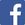300000

English | Français | فارسی | Українська | Azerbaijani

### Induction Proof Builder

Instructions

Start by reading the overview below to learn how proof by induction works or to get a refresher.

Select a mode on the form below, then select a proof by induction problem and click 'Start' to begin.

Modes:

• Question: Perform the proof by induction yourself.
• Example: Watch a proof by induction performed for the chosen problem.

If you get stuck on a line in the proof, try the help button [?]. It will display a help section specific to the current part of the proof, and may have hints on what you need to do next.

Hovering the mouse over a button will give a description of what it does and how to use it.

The fastest way to master the interface is to open two browser windows, one with a problem in 'Example' mode and one with the same problem in 'Question' mode. By going through the example step-by-step in one window, you can try each step in the other window at the same time.

Mode: Problem:
01000.Sum of Integers.json|01005.Sum of (3i - 2).json|01010.Sum of Halves.json|01015.Sum of Squares.json|01016.Sum of Cubes.json|01020.Sum of Odd Integers.json|01025.Sum of Products of Sequential Integers.json|01030.Sum of Squares of Even Integers.json|01031.Sum of Squares of Odd Integers.json|01035.Sum of Squares with Alternating Signs.json|01050.Sum of Powers of Two.json|01051.Sum of Powers of Three.json|01052.Sum of Powers of Four.json|01054.Sum of (i + 1) (2 ^ i).json|01055.Geometric Series.json|01400.Sum of 1 over i (i + 1).json|01420.Sum of 1 over (2i - 1) (2i + 1).json|01500.Sum of 1, 2, ..., n, ..., 2, 1.json|02010.Product of Sequence of i over (i + 1).json|02020.Product of Sequence of (i - 1) over (i + 1).json|02050.Product of Sequence of (i^2 - 1) over (i^2).json|03006.Divisibility of (5^n - 2^n) by 3.json|03017.Divisibility of (7^n - 3^n) by 4.json|03020.Divisibility of (a^n - 1) by (a - 1).json|03021.Divisibility of (a^n - b^n) by (a - b).json|03022.Divisibility of (11^n - 6) by 5.json|03024.Divisibility of (n^2 - n) by 2.json|03025.Divisibility of (n^3 - n) by 3.json|03026.Divisibility of (n^3 + 2n) by 3.json|03030.Divisibility of ((2n - 1)^2 - 1) by 8.json|03033.Divisibility of (3^2n - 1) by 8.json|03035.Divisibility of (10^(n+1) + 10^n + 1) by 3.json|03040.Divisibility of (5^n + 2 (11^n)) by 3.json|03050.Divisibility of (n (n^2 +5)) by 3.json|03055.Divisibility of (n^3 + (n+1)^3 + (n+2)^3) by 9.json|03060.Divisibility of (2 ^ (2n) - 1) by 3.json|03065.Divisibility of (2^(n+2) + 3^(2n+1)) by 7.json|03070.Divisibility of (2 (n^3) + 3 (n^2) + n) by 6.json|03073.Divisibility of (3 (5^(2n+1)) + 2^(3n+1)) by 17.json|03075.Divisibility of (5^(2n + 1) + 2^(2n + 1)) by 7.json|04000.Sum of Fibonacci Terms.json|04001.Sum of Even Fibonacci Terms.json|04002.Sum of Odd Fibonacci Terms.json|04010.Sum of (Fibonacci(i) ^ 2).json|04050.Sum of (Fibonacci(i) ^ 2) (Fibonacci(i + 1)).json|04100.((Fibonacci(n+1)) (Fibonacci(n-1)) - Fibonacci(n)^2) Identity.json|
01000.Sum of Integers.json|01005.Sum of (3i - 2).json|01010.Sum of Halves.json|01015.Sum of Squares.json|01016.Sum of Cubes.json|01020.Sum of Odd Integers.json|01025.Sum of Products of Sequential Integers.json|01030.Sum of Squares of Even Integers.json|01031.Sum of Squares of Odd Integers.json|01035.Sum of Squares with Alternating Signs.json|01050.Sum of Powers of Two.json|01051.Sum of Powers of Three.json|01052.Sum of Powers of Four.json|01054.Sum of (i + 1) (2 ^ i).json|01055.Geometric Series.json|01400.Sum of 1 over i (i + 1).json|01420.Sum of 1 over (2i - 1) (2i + 1).json|01500.Sum of 1, 2, ..., n, ..., 2, 1.json|02010.Product of Sequence of i over (i + 1).json|02020.Product of Sequence of (i - 1) over (i + 1).json|02050.Product of Sequence of (i^2 - 1) over (i^2).json|03006.Divisibility of (5^n - 2^n) by 3.json|03017.Divisibility of (7^n - 3^n) by 4.json|03020.Divisibility of (a^n - 1) by (a - 1).json|03021.Divisibility of (a^n - b^n) by (a - b).json|03022.Divisibility of (11^n - 6) by 5.json|03024.Divisibility of (n^2 - n) by 2.json|03025.Divisibility of (n^3 - n) by 3.json|03026.Divisibility of (n^3 + 2n) by 3.json|03030.Divisibility of ((2n - 1)^2 - 1) by 8.json|03033.Divisibility of (3^2n - 1) by 8.json|03035.Divisibility of (10^(n+1) + 10^n + 1) by 3.json|03040.Divisibility of (5^n + 2 (11^n)) by 3.json|03050.Divisibility of (n (n^2 +5)) by 3.json|03055.Divisibility of (n^3 + (n+1)^3 + (n+2)^3) by 9.json|03060.Divisibility of (2 ^ (2n) - 1) by 3.json|03065.Divisibility of (2^(n+2) + 3^(2n+1)) by 7.json|03070.Divisibility of (2 (n^3) + 3 (n^2) + n) by 6.json|03073.Divisibility of (3 (5^(2n+1)) + 2^(3n+1)) by 17.json|03075.Divisibility of (5^(2n + 1) + 2^(2n + 1)) by 7.json|04000.Sum of Fibonacci Terms.json|04001.Sum of Even Fibonacci Terms.json|04002.Sum of Odd Fibonacci Terms.json|04010.Sum of (Fibonacci(i) ^ 2).json|04050.Sum of (Fibonacci(i) ^ 2) (Fibonacci(i + 1)).json|04100.((Fibonacci(n+1)) (Fibonacci(n-1)) - Fibonacci(n)^2) Identity.json|